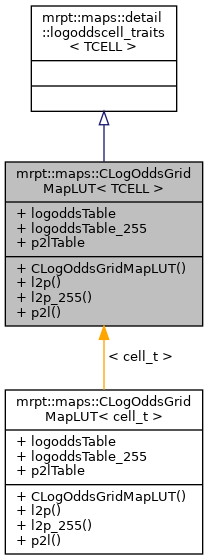MRPT  1.9.9
mrpt::maps::CLogOddsGridMapLUT< TCELL > Struct Template Reference

## Detailed Description

### template<typename TCELL> struct mrpt::maps::CLogOddsGridMapLUT< TCELL >

One static instance of this struct should exist in any class implementing CLogOddsGridMap2D to hold the Look-up-tables (LUTs) for log-odss Bayesian update.

Map cells must be type TCELL, which can be only:

• int8_t or
• int16_t
CLogOddsGridMap2D, see derived classes for usage examples.

Definition at line 157 of file CLogOddsGridMap2D.h.

#include <mrpt/maps/CLogOddsGridMap2D.h>

Inheritance diagram for mrpt::maps::CLogOddsGridMapLUT< TCELL >:## Public Types

using cell_t = TCELL
The type of. More...

using traits_t = detail::logoddscell_traits< TCELL >

## Public Member Functions

CLogOddsGridMapLUT ()
Constructor: computes all the required stuff. More...

float l2p (const cell_t l)
Scales an integer representation of the log-odd into a real valued probability in [0,1], using p=exp(l)/(1+exp(l)) More...

uint8_t l2p_255 (const cell_t l)
Scales an integer representation of the log-odd into a linear scale [0,255], using p=exp(l)/(1+exp(l)) More...

cell_t p2l (const float p)
Scales a real valued probability in [0,1] to an integer representation of: log(p)-log(1-p) in the valid range of cell_t. More...

## Public Attributes

std::vector< float > logoddsTable
A lookup table to compute occupancy probabilities in [0,1] from integer log-odds values in the cells, using. More...

std::vector< uint8_tlogoddsTable_255
A lookup table to compute occupancy probabilities in the range [0,255] from integer log-odds values in the cells, using. More...

std::vector< cell_tp2lTable
A lookup table for passing from float to log-odds as cell_t. More...

## ◆ cell_t

template<typename TCELL>
 using mrpt::maps::CLogOddsGridMapLUT< TCELL >::cell_t = TCELL

The type of.

Definition at line 160 of file CLogOddsGridMap2D.h.

## ◆ traits_t

template<typename TCELL>
 using mrpt::maps::CLogOddsGridMapLUT< TCELL >::traits_t = detail::logoddscell_traits

Definition at line 161 of file CLogOddsGridMap2D.h.

## ◆ CLogOddsGridMapLUT()

template<typename TCELL>
 mrpt::maps::CLogOddsGridMapLUT< TCELL >::CLogOddsGridMapLUT ( )
inline

Constructor: computes all the required stuff.

Definition at line 180 of file CLogOddsGridMap2D.h.

## ◆ l2p()

template<typename TCELL>
 float mrpt::maps::CLogOddsGridMapLUT< TCELL >::l2p ( const cell_t l )
inline

Scales an integer representation of the log-odd into a real valued probability in [0,1], using p=exp(l)/(1+exp(l))

Definition at line 220 of file CLogOddsGridMap2D.h.

## ◆ l2p_255()

template<typename TCELL>
 uint8_t mrpt::maps::CLogOddsGridMapLUT< TCELL >::l2p_255 ( const cell_t l )
inline

Scales an integer representation of the log-odd into a linear scale [0,255], using p=exp(l)/(1+exp(l))

Definition at line 232 of file CLogOddsGridMap2D.h.

## ◆ p2l()

template<typename TCELL>
 cell_t mrpt::maps::CLogOddsGridMapLUT< TCELL >::p2l ( const float p )
inline

Scales a real valued probability in [0,1] to an integer representation of: log(p)-log(1-p) in the valid range of cell_t.

Definition at line 244 of file CLogOddsGridMap2D.h.

## ◆ logoddsTable

template<typename TCELL>
 std::vector mrpt::maps::CLogOddsGridMapLUT< TCELL >::logoddsTable

A lookup table to compute occupancy probabilities in [0,1] from integer log-odds values in the cells, using.

Definition at line 167 of file CLogOddsGridMap2D.h.

## ◆ logoddsTable_255

template<typename TCELL>
 std::vector mrpt::maps::CLogOddsGridMapLUT< TCELL >::logoddsTable_255

A lookup table to compute occupancy probabilities in the range [0,255] from integer log-odds values in the cells, using.

This is used to speed-up conversions to grayscale images.

Definition at line 174 of file CLogOddsGridMap2D.h.

## ◆ p2lTable

template<typename TCELL>
 std::vector mrpt::maps::CLogOddsGridMapLUT< TCELL >::p2lTable

A lookup table for passing from float to log-odds as cell_t.

Definition at line 177 of file CLogOddsGridMap2D.h.

 Page generated by Doxygen 1.8.14 for MRPT 1.9.9 Git: 7d5e6d718 Fri Aug 24 01:51:28 2018 +0200 at lun nov 2 08:35:50 CET 2020